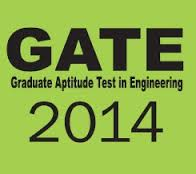## September 5, 2013

GATE Syllabus for Civil Engineering (CE) 2014 PDF Download : 1. Civil Engineering stream is provided only by some engineering colleges, so in order to gain better knowledge in this field candidates plan for their masters.2. M.Tech is one of the post graduation course which is taken by most of the Civil Engineering students. All these candidates must appear for GATE exam to get admission in any college.

3. Look at the syllabus for Civil Engineering in GATE-2014 exam

4. ENGINEERING MATHEMATICS
Linear Algebra: Matrix algebra, Systems of linear equations, Eigen values and eigen vectors.

Calculus: Functions of single variable, Limit, continuity and differentiability, Mean value theorems, Evaluation of definite and improper integrals, Partial derivatives, Total derivative, Maxima and minima, Gradient, Divergence and Curl, Vector identities, Directional derivatives, Line, Surface and Volume integrals, Stokes, Gauss and Green’s theorems.

Differential equations: First order equations (linear and nonlinear), Higher order linear differential equations with constant coefficients, Cauchy’s and Euler’s equations, Initial and boundary value problems, Laplace transforms, Solutions of one dimensional heat and wave equations and Laplace equation.

Complex variables: Analytic functions, Cauchy’s integral theorem, Taylor and Laurent series.

Probability and Statistics: Definitions of probability and sampling theorems, Conditional probability, Mean, median, mode and standard deviation, Random variables, Poisson, Normal and Binomial distributions.

Numerical Methods: Numerical solutions of linear and non-linear algebraic equations Integration by trapezoidal and Simpson’s rule, single and multi-step methods for differential equations.

5. STRUCTURAL ENGINEERING
Mechanics: Bending moment and shear force in statically determinate beams. Simple stress and strain relationship: Stress and strain in two dimensions, principal stresses, stress transformation, Mohr’s circle. Simple bending theory, flexural and shear stresses, unsymmetrical bending, shear centre. Thin walled pressure vessels, uniform torsion, buckling of column, combined and direct bending stresses.

Structural Analysis: Analysis of statically determinate trusses, arches, beams, cables and frames, displacements in statically determinate structures and analysis of statically indeterminate structures by force/ energy methods, analysis by displacement methods (slope deflection and moment distribution methods), influence lines for determinate and indeterminate structures. Basic concepts of matrix methods of structural analysis.

Concrete Structures: Concrete Technology- properties of concrete, basics of mix design. Concrete design- basic working stress and limit state design concepts, analysis of ultimate load capacity and design of members subjected to flexure, shear, compression and torsion by limit state methods. Basic elements of prestressed concrete, analysis of beam sections at transfer and service loads.

Steel Structures: Analysis and design of tension and compression members, beams and beam- columns, column bases. Connections- simple and eccentric, beam–column connections, plate girders and trusses. Plastic analysis of beams and frames.

6. GEOTECHNICAL ENGINEERING
Soil Mechanics: Origin of soils, soil classification, three-phase system, fundamental definitions, relationship and interrelationships, permeability &seepage, effective stress principle, consolidation, compaction, shear strength.

Foundation Engineering: Sub-surface investigations- scope, drilling bore holes, sampling, penetration tests, plate load test. Earth pressure theories, effect of water table, layered soils. Stability of slopes-infinite slopes, finite slopes. Foundation types-foundation design requirements. Shallow foundations-bearing capacity, effect of shape, water table and other factors, stress distribution, settlement analysis in sands & clays. Deep foundations–pile types, dynamic & static formulae, load capacity of piles in sands & clays, negative skin friction.

7. WATER RESOURCES ENGINEERING
Fluid Mechanics and Hydraulics: Properties of fluids, principle of conservation of mass, momentum, energy and corresponding equations, potential flow, applications of momentum and Bernoulli’s equation, laminar and turbulent flow, flow in pipes, pipe networks. Concept of boundary layer and its growth. Uniform flow, critical flow and gradually varied flow in channels, specific energy concept, hydraulic jump. Forces on immersed bodies, flow measurements in channels, tanks and pipes. Dimensional analysis and hydraulic modeling. Kinematics of flow, velocity triangles and specific speed of pumps and turbines.

Hydrology: Hydrologic cycle, rainfall, evaporation, infiltration, stage discharge relationships, unit hydrographs, flood estimation, reservoir capacity, reservoir and channel routing. Well hydraulics.

Irrigation: Duty, delta, estimation of evapo-transpiration. Crop water requirements. Design of: lined and unlined canals, waterways, head works, gravity dams and spillways. Design of weirs on permeable foundation. Types of irrigation system, irrigation methods. Water logging and drainage, sodic soils.

8. ENVIRONMENTAL ENGINEERING
Water requirements: Quality standards, basic unit processes and operations for water treatment. Drinking water standards, water requirements, basic unit operations and unit processes for surface water treatment, distribution of water. Sewage and sewerage treatment, quantity and characteristics of waste water. Primary, secondary and tertiary treatment of waste water, sludge disposal, effluent discharge standards. Domestic waste water treatment, quantity of characteristics of domestic waste water, primary and secondary treatment Unit operations and unit processes of domestic waste water, sludge disposal.

Air Pollution: Types of pollutants, their sources and impacts, air pollution meteorology, air pollution control, air quality standards and limits.

Municipal Solid Wastes: Characteristics, generation, collection and transportation of solid wastes, engineered systems for solid waste management (reuse/ recycle, energy recovery, treatment and disposal).

Noise Pollution: Impacts of noise, permissible limits of noise pollution, measurement of noise and control of noise pollution.

9. TRANSPORTATION ENGINEERING
Highway Planning: Geometric design of highways, testing and specifications of paving materials, design of flexible and rigid pavements.

Traffic Engineering: Traffic characteristics, theory of traffic flow, intersection design, traffic signs and signal design, highway capacity.

10. SURVEYING
Importance of surveying, principles and classifications, mapping concepts, coordinate system, map projections, measurements of distance and directions, leveling, theodolite traversing, plane table surveying, errors and adjustments, curves.

11. Visit the official website of GATE-2014 for more details. http://gate.iitkgp.ac.in/gate2014/